[HomePage] Prof W.W.Sawyer

### ALGEBRA, THE CEMENT OF MATHEMATICS.

W. W. Sawyer.

Computers are rapidly making it possible for any purely mechanical mathematical operation to be carried out by a suitable program. This will raise an interesting problem for the learning and teaching of mathematics. In the past -say 70 years ago - young mathematicians became proficient in the mechanical processes of algebra by practising them (sometimes with boring and excessive drill) and in the course of this achieved some feeling for the strategy of algebra, how operations could be combined to give new and interesting results. Basically algebra enables us to derive from some collection of results, expressed by equations, other equations which may be far from obvious. How this feeling for algebraic possibilities is to be conveyed in the computer age is a question of increasing importance.

This article does not attempt to solve that problem. Its aim is simply to give an example of the way in which algebra can tie a new result to theorems we already know.

There is a well-known formula for the area of a triangle in terms of its sides It is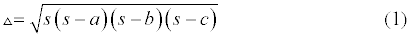where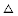is the area of the triangle, and s is the semi-perimeter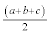. It is natural to wonder where this result comes from and how it is obtained. The only device needed is a result discussed by Jack Forster in the April 1992 issue of this magazine,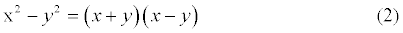conveniently memorized by the words "difference of squares equals sum times difference".

We start from two known results. The area of a triangle being half the base times the height, we have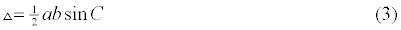We suppose we have been given the sides a,b,c, so the angle C in equation (2) is something we have to find. Probably the simplest and best known formula for this purpose is the cosine rule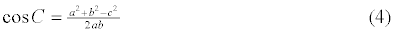Unfortunately this formula gives us the cosine and not the sine. We need one more equation, which Pythagoras Theorem gives us,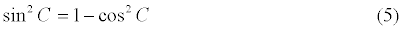As 1 is a perfect square, we can apply our equation (2),

with x = 1 and y = cosC. This gives us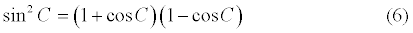We shall have another chance to use equation (2) when we look more closely at the brackets, (1 + cosC) and (1 - cosC) .

If we add 1 to each side in equation (4), and put all the right-hand side over the denominator 2ab we find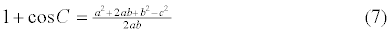We now notice that a2 + 2ab + b2 is a perfect square, being in fact (a + b)2, so the numerator in equation (7) is a difference of squares, and we may write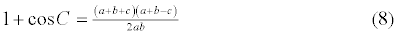We now do a similar trick with 1 - cosC. Using formula (4) for cosC, and again putting everything over the denominator 2ab, at first we obtain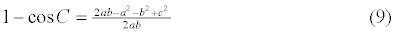It is not immediately obvious that the numerator here too is a difference of squares, but on reflection it will be seen that it is in fact c2 - (a - b)2 so we have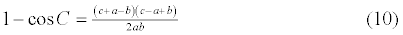If we multiply equation (8) by equation (10), we find on the left-hand side

(1 + cosC)(1 - cosC), which by equation (6) is sin2C. Equation (1), the result we are trying to prove has everything under a square-root sign; accordingly it is sensible to consider the square of the area, which, by equation (3), is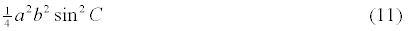and this ought to be s(s - a)(s - b)(s - c). All we have to do now is to use the meaning of s; it is. When we multiply equation (8) by equation (10) we get 4 factors on the right-hand side, and these in fact are very close to what we hoped they would be,
for
a + b +c = 2s, a + b - c = 2s - 2c and so on. All that remains to be done is to cancel factors involving a, b and c.

You will probably agree it is far from obvious that equation (1) is a consequence of equation (3). Algebra has allowed us to explain the connection. In fact algebra does rather more than this. If at some time in the past it became necessary to find a formula for the area of a triangle in terms of the sides, the steps taken above would seem extremely natural to someone fairly familiar with work in traditional elementary algebra, and would indeed lead to the discovery of the desired formula.

From Nov 1992 issue (Vol. 3, No. 2) Mathematical Review
(VI form magazine,) Warwick University.